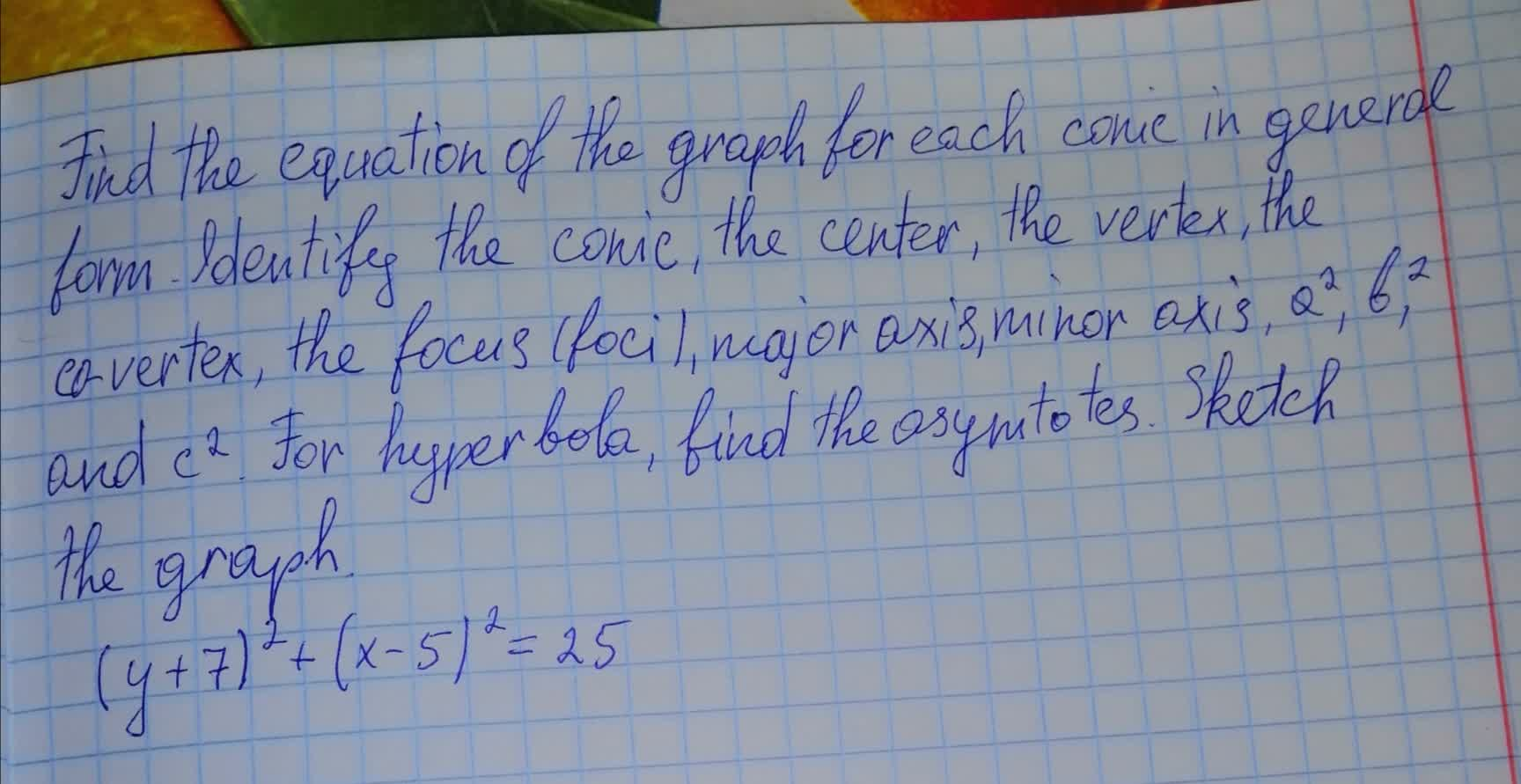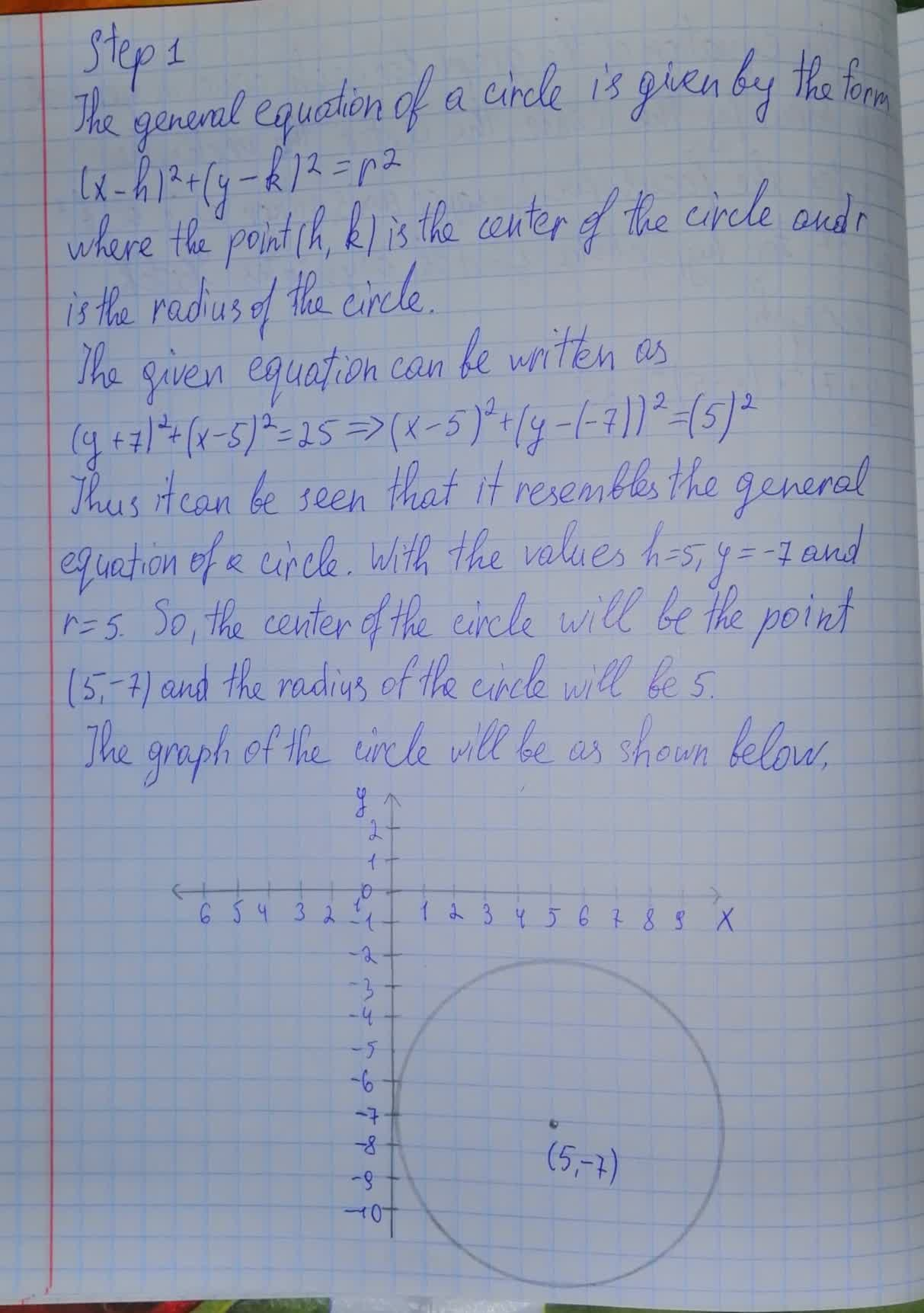Find the equation of the graph for each conic in general form. For hyperbola,find the asymtotes.Sketch the graph (y+7)^2+(x-5)^2=25Trent Carpenter 2021-08-08 Answered
Find the equation of the graph for each conic in general form. Identify the conic, the center, the vertex, the co-vertex, the focus (foci), major axis, minor axis, $$\displaystyle{a}^{{2}}$$, $$\displaystyle{b}^{{2}}$$, and $$\displaystyle{c}^{{2}}$$. For hyperbola,find the asymtotes.Sketch the graph
$$\displaystyle{\left({y}+{7}\right)}^{{2}}+{\left({x}-{5}\right)}^{{2}}={25}$$• Questions are typically answered in as fast as 30 minutes

Solve your problem for the price of one coffee

• Math expert for every subject
• Pay only if we can solve itViktor Wiley